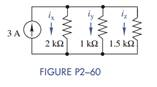Create an Account

Home / Questions / Use current division in Figure P2−60 to find i x , i y , and i z . Then show that the sum of...

Use current division in Figure P2−60 to find i x , i y , and i z . Then show that the sum of...

Use current division in Figure P2−60 to find ix, iy, and iz. Then show that the sum of these currents equals the source current.Jul 28 2020 View more View LessSubscribe To Get Solution